# Getting Started with SPICE OPUS

Now we can run Spice Opus program (Windows)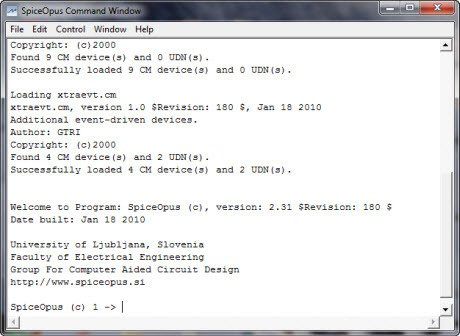Let us consider a BJT amplifier in the common emitter configuration with an emitter capacitor as in the image below (The schematic is not designed with spice opus that doesn’t have a built-in schematic program)We have to edit a .cir file that describes this circuit. Let’s open a text editor, for example the Notepad, and begin to describe the circuit. The netlist of the example above is the following:

BJT common emitter configuration with emitter capacitor

* Biasing Supply

vcc vcc 0 dc 12

* Input Signal Source

vin in 0 dc 0 ac 1mv 0 sin(0 1mv 10kHz)

* Biasing Resistors

r1 vcc b 9.8k

r2 b 0 2.2k

re e 0 300

rc vcc c 900

* Coupling Capacitors

cin in b 4.7uf

cout c out 10uf

* Emitter Capacitor

ce e 0 100uf

rout out 0 47k

* Transistor

q c b e BC368

* model of Transistor BC368

.model BC368    NPN(Is=14.06f Xti=3 Eg=1.11 Vaf=100 Bf=187.6 Ise=137.2f

+               Ne=1.468 Ikf=4.103 Xtb=1.5 Br=4.541 Isc=44.13f

+               Nc=1.471 Ikr=1.701 Rc=91m Cjc=52.24p Mjc=.3333 Vjc=.5 Fc=.5

+               Cje=156p Mje=.3333 Vje=.5 Tr=10n Tf=964.8p Itf=595.3

+               Xtf=1.001K Vtf=10)

.end

It’s important rename the file *.txt as *.cir, for this example assign the name BJTcommonemitter.cir. Add the file in the installation directory, in our case C:Spiceopus. Now let’ s load the netlist of the circuit into the simulator with the “source” command: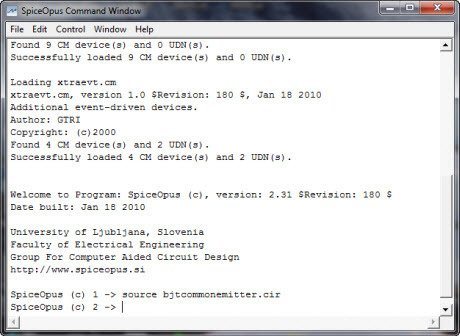The filename is case insensitive. A transient analysis from 0 to 1000us with a 10kHz sine wave input is performed by “tran” command, with a step of 1us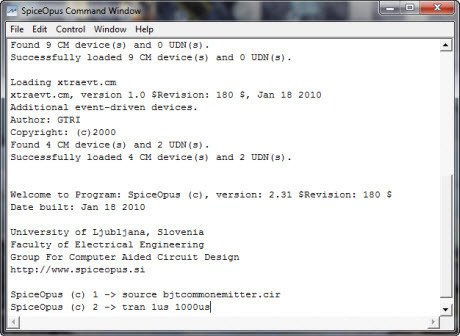The results of analysis can be plotted by “plot” command.Here we specify vout, vin *50, the labels of x and y axes, and time in ms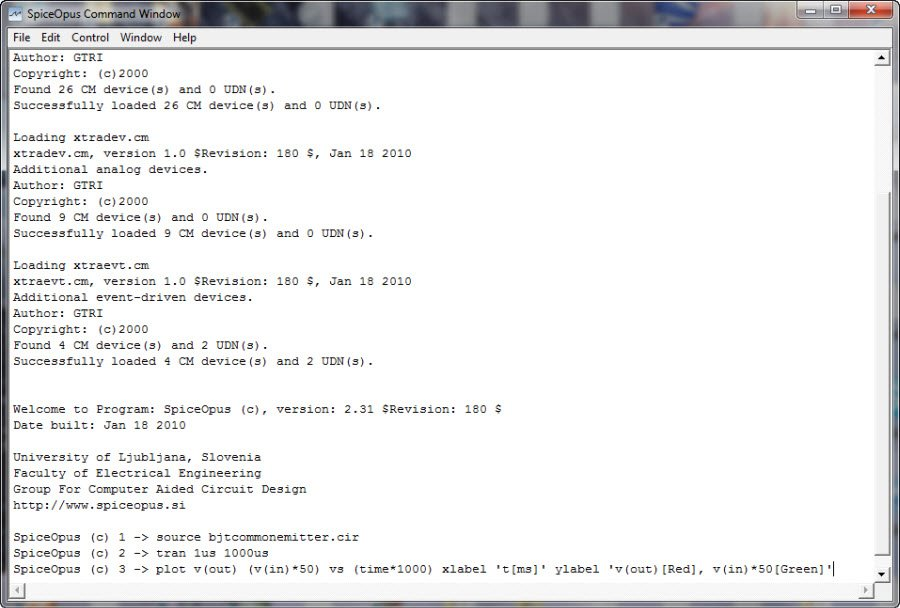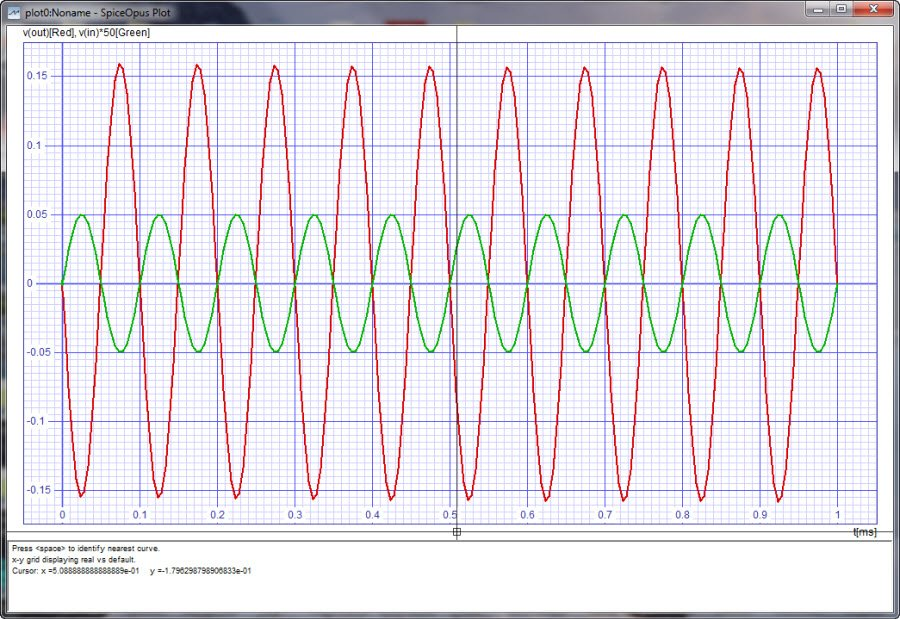Posted in SPICE OPUS and tagged , , , , , .